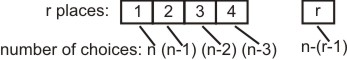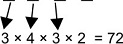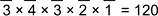# The number of integers greater than 6,000 that can be formed, using the digits 3, 5, 6,7 and 8, without repetition, is : Option 1) 216 Option 2) 192 Option 3) 120 Option 4) 72

D Divya Saini

As we learnt in

Number of Permutations without repetition -

Arrange n objects taken r at a time equivalent to filling r places from n things.- wherein

Where

Four digit numbers can beFive digit numbersTotal = 192

Correct option is 2.

Option 1)

216

This is an incorrect option.

Option 2)

192

This is the correct option.

Option 3)

120

This is an incorrect option.

Option 4)

72

This is an incorrect option.

Exams
Articles
Questions